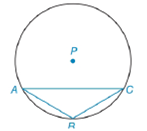Chapter 6.4, Problem 38E### Elementary Geometry for College St...

6th Edition
Daniel C. Alexander + 1 other
ISBN: 9781285195698

#### Solutions

Chapter
Section### Elementary Geometry for College St...

6th Edition
Daniel C. Alexander + 1 other
ISBN: 9781285195698
Textbook Problem
1 views

# In ⊙ P , whose radius has length 8 i n . , m A B ⌢ = m B C ⌢ = 60 ° . Because m A C ⌢ = 120 ° chord A C ¯ is longer than either of the congruent chords A B ¯ and B C ¯ . Determine how much longer A C ¯ is than A B ¯ ; that is, find the exact value and the approximate value of A C − A B .To determine

To find:

The exact value and the approximate value of ACAB.

Explanation

Given:

P, whose radius has length 8 in., mAB=mBC=60°. mAC=120° and chord AC¯ is longer than either of the congruent chords AB¯ and BC¯.

The figure given below,

Property Used:

The measure of an inscribed angle of a circle is half the measure of its intercepted arc. Consider the figure below, let the inscribed angle be 2.

Then m2=12mHJ and generally HKKJ.

According to the Pythagorean theorem, in a right-angled triangle

hypotenuse2=base2+perpendicular2.

Calculation:

The measure of an inscribed angle of a circle is half the measure of its intercepted arc and from the given figure,

mCAB=12mBC=12(60°)=30°

mBCA=12mAB=12(60°)=30° and

mCBA=12(360°mAC)=12(360°120°)=12(240°)=120°.

Since, two angles are equal in the triangle, hence it is an isosceles triangle. So, the two sides corresponding legs will be equal i.e. AB¯=BC¯.

Let L, M, and N be perpendicular bisector on chords AB¯, BC¯ and AC¯ respectively as a radius perpendicular to a chord bisects the chord and its arc.

Since, radii are equal AP=PB we get the angles also equal i.e.

PAB=PBA=180°60°2=60°

Also, APB=mAB=60°. Since all angles are equal, APB is equilateral triangle.

Thus, mPB¯=mPA¯=mAB¯=8.

Similarly, radii are equal PB=PC we get the angles also equal i.e.

PBC=PCB=180°60°2=60°

Also, BPC=mBC=60°

### Still sussing out bartleby?

Check out a sample textbook solution.

See a sample solution

#### The Solution to Your Study Problems

Bartleby provides explanations to thousands of textbook problems written by our experts, many with advanced degrees!

Get Started

#### In Exercises 31-34, evaluate h(2), where h = g f. 34. f(x)=1x1;g(x)=x2+1

Applied Calculus for the Managerial, Life, and Social Sciences: A Brief Approach

#### Solve the equations in Exercises 126. x1x=0

Finite Mathematics and Applied Calculus (MindTap Course List)

#### If 13f(x)dx=10 and 13g(x)dx=6, then 13(2f(x)3g(x))dx= a) 2 b) 4 c) 18 d) 38

Study Guide for Stewart's Single Variable Calculus: Early Transcendentals, 8th